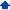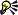• Bessel — These spreadsheet functions return values that satisfy the Bessel equation or the modified Bessel equation. Bessel functions have various applications in physics and engineering.
 • Boolean — These spreadsheet functions handle applications of digital logic and bitwise operations, which involve the testing, setting, or shifting of actual bits in a number.
 • Complex number — These spreadsheet functions convert or modify a complex number (a number whose square is a negative real number).
 • Miscellaneous — These spreadsheet functions have various applications in engineering, including converting values to different measures, testing the relationship of two numeric values, and returning error functions.
 • Number conversion — These spreadsheet functions convert a value from one number system to another.• @BESSELI Returns the modified Bessel function In(x).
 • @BESSELJ Returns the Bessel function Jn(x).
 • @BESSELK Returns the modified Bessel function Kn(x).
 • @BESSELY Returns the Bessel function Yn(x).• @ADDB Returns the sum of two binary numbers.
 • @ADDBO Returns the overflow bit of the sum of two binary numbers.
 • @ADDHO Returns the overflow bit of the sum of two hexadecimal numbers.
 • @ANDB Returns the AND operation of two binary numbers.
 • @ANDH Returns the AND operation of two hexadecimal numbers.
 • @BITRB Returns a binary number with a specified bit reset to 0.
 • @BITRH Returns a hexadecimal number with a specified bit reset to 0.
 • @BITSB Returns a binary number with a specified bit set to 1.
 • @BITSH Returns a hexadecimal number with a specified bit set to 1.
 • @BITTB Returns the value of a specified bit in a binary number.
 • @BITTH Returns the value of a specified bit in a hexadecimal number.
 • @CATB Returns the concatenation of two binary numbers.
 • @CATH Returns the concatenation of two hexadecimal numbers.
 • @CATNB Returns the concatenation of n binary numbers.
 • @CATNH Returns the concatenation of n hexadecimal numbers.
 • @INVB Returns the inverse of a binary number.
 • @INVH Returns the inverse of a hexadecimal number.
 • @ORB Returns the OR operation of binary numbers.
 • @ORH Returns the OR operation of hexadecimal numbers.
 • @SHLB Returns a binary number shifted left by a specified number of bits.
 • @SHLBO Returns the overflow bit of a binary number after it has been shifted left one bit.
 • @SHLH Returns a hexadecimal number shifted left by a specified number of bits.
 • @SHLHO Returns the overflow bit of a hexadecimal number after it has been shifted left one bit.
 • @SHRB Returns a binary number shifted right by a specified number of bits.
 • @SHRBO Returns the overflow bit of a binary number after it has been shifted right one bit.
 • @SHRH Returns a hexadecimal number shifted right by a specified number of bits.
 • @SHRHO Returns the overflow bit of a hexadecimal number after it has been shifted right one bit.
 • @SUBB Returns the difference of two binary numbers.
 • @SUBBO Returns the overflow bit of the subtraction of one binary number from another.
 • @SUBH Returns the difference of two binary numbers.
 • @SUBHO Returns the overflow bit of the subtraction of one hexadecimal number from another.
 • @XORB Returns the exclusive OR operation of binary numbers.
 • @XORH Returns the exclusive OR operation of hexadecimal numbers.

##### Entering Boolean functions

The shift @functions, @SHLB, @SHRB, @SHLH, and @SHRH, shift the bits in a number to the left or right. You can use the shift @functions to perform quick multiplication of integers. Each binary shift to the left is equivalent to multiplying the number by 2. Each binary shift to the right is equivalent to dividing the number by 2.

 Binary Decimal 00011 3 00110 6 01100 12 11000 24

As you shift bits off one end of a number, bits are added to the other end. Shift, however, is not equal to rotate. For example, the binary number 1001 shifted left is 0010. The same number rotated left is 0011. To perform this rotation, you can use a nested bit test @function to set the BitIn argument.

The addition @functions, @ADDB and @ADDH, return the sum of two numbers. The subtraction @functions, @SUBB and @SUBH, return the difference of two numbers.

The overflow @functions, @SHLBO, @SHRBO, @SHLHO, @SHRHO, @ADDBO, @ADDHO, @SUBBO, @SUBHO, return the overflow bit (either 0 or 1) of a shift, addition, or subtraction operation. For example, if you shift the binary
number 1001 to the left and maintain four places, the result is 0010. The overflow bit, that is, the bit that has shifted into the fifth place not shown, is 1.

The AND @functions, @ANDB and @ANDH, combine the zeros of the input words. Any bit that is 0 in either number sets the corresponding output bit to 0.

The OR @functions, @ORB and @ORH, combine the ones of the input words. Any bit that is 1 in either number sets the corresponding output bit to 1.

The exclusive OR @functions, @XORB and @XORH, set an output bit to 1 when two corresponding input bits are not equal.

The following table shows the results of AND, OR, and exclusive OR operations of Bit A and Bit B.

 Bit A Bit B A AND B A OR B A XOR B 0 0 0 0 0 0 1 0 1 1 1 0 0 1 1 1 1 1 1 0

The invert @functions, @INVB and @INVH, invert individual bits of a number; that is, all bits with a value of 1 change to 0, and all bits with a value of 0 change to 1.

The bit manipulation @functions, @BITSB, @BITRB, @BITTB, @BITSH, @BITRH, and @BITTH, let you set or reset bits, or test the value of a bit in a number.

The concatenation @functions, @CATB, @CATH, @CATNB, and @CATNH, link numbers together in a chain. For example, the concatenation of the two binary numbers 11111 and 10101 is 1111110101.

The argument Bits defines the word size (in number of binary bits) for both input and output. The default for Bits is the number of bits in the largest input number. If you perform an operation with two numbers of different word size, the smaller number is left-padded with zeros. If Bits is less than the length of an input value, then the excess most significant digits are truncated. For Boolean @functions using hexadecimal numbers, note that each hexadecimal digit equals 4 bits.

The argument BitIn represents either the binary bit inserted during a shift, the carry bit for addition, or the borrow bit for subtraction; it can be either 0 (the default) or 1.• To add or subtract negative numbers with the Boolean @functions, use two’s complement notation. Two’s complement notation is a code used to give meaning to a binary string. The sign bit of two’s complement notation is the leftmost bit of the word. A number is positive if the sign bit is 0 and negative if it is 1. Negative integers are converted to two’s complement by inverting each bit (that is, changing each 1 to a 0 and each 0 to a 1), and then adding 1.

#### Complex Number Engineering spreadsheet functions• @COMPLEX Converts real & imaginary coefficients into a complex number.
 • @IMABS Returns the distance from the origin on a complex plane for a complex number.
 • @IMAGINARY Returns the imaginary coefficient of a complex number.
 • @IMARGUMENT Returns the argument theta, an angle expressed in radians.
 • @IMCONJUGATE Returns the complex conjugate of a complex number.
 • @IMCOS Returns the cosine of a complex number.
 • @IMDIV Returns the quotient of two complex numbers.
 • @IMEXP Returns the exponential of a complex number.
 • @IMLN Returns the natural logarithm of a complex number.
 • @IMLOG10 Returns the base-10 logarithm of a complex number.
 • @IMLOG2 Returns the base-2 logarithm of a complex number.
 • @IMPOWER Returns a complex number raised to a complex power.
 • @IMPRODUCT Returns the product of two complex numbers.
 • @IMREAL Returns the real coefficient of a complex number.
 • @IMSIN Returns the sine of a complex number.
 • @IMSQRT Returns the square root of a complex number.
 • @IMSUB Returns the difference of two complex numbers.
 • @IMSUM Returns the sum of complex numbers.• @DELTA Tests whether two numbers are equal.
 • @ERF Returns the error function.
 • @ERFC Returns the complementary function.
 • @ERFD Returns the derivative of the error function.
 • @GAMMA Calculates the gamma distribution function.
 • @GESTEP Tests whether a number is greater than a threshold value.
 • @SPLINE Returns a polynomial fitted piecewise to pass through a specified set of points.
 • @USESPLINE Returns, for any specified x value, the corresponding y value, specified the original t and y arrays and the coefficient array produced by @SPLINE.

#### Number Conversion Engineering spreadsheet functions• @ASCTOHEX Returns the hexadecimal string equivalent of an ASCII value
 • @BASE Converts a base-10 number into another base.
 • @BINTOHEX Converts a binary number to hexadecimal.
 • @BINTOHEX64 Converts a binary number (up to 64 bits) to hexadecimal.
 • @BINTONUM Converts a binary number to decimal.
 • @BINTONUM64 Converts a binary number (up to 64 bits) to decimal.
 • @BINTOOCT Converts a binary number to octal.
 • @BINTOOCT64 Converts a binary number (up to 64 bits) to octal.
 • @CONVERT Converts a number from one measurement system to another.
 • @HEXTOASC Converts a hexadecimal number to ASCII.
 • @HEXTOBIN Converts a hexadecimal number to binary.
 • @HEXTOBIN64 Converts a hexadecimal number (up to 64 bits) to binary.
 • @HEXTONUM  Converts a hexadecimal number to decimal.
 • @HEXTONUM64 Converts a hexadecimal number (up to 64 bits) to decimal.
 • @HEXTOOCT Converts a hexadecimal number to octal.
 • @HEXTOOCT64 Converts a hexadecimal number (up to 64 bits) to octal.
 • @NUMTOBIN Converts a decimal number to binary.
 • @NUMTOBIN64 Converts a decimal number (up to 64 bits) to binary.
 • @NUMTOHEX  Converts a decimal number to hexadecimal.
 • @NUMTOHEX64 Converts a decimal number (up to 64 bits) to hexadecimal.
 • @NUMTOOCT Converts a decimal number to octal.
 • @NUMTOOCT64 Converts a decimal number (up to 64 bits) to octal.
 • @OCTTOBIN Coverts an octal number to binary.
 • @OCTTOHEX Converts an octal number to hexadecimal.
 • @OCTTONUM Converts an octal number to decimal.

##### Entering Number Conversion functions

Input numbers that include non-numeric characters (such as hexadecimal or ASCII values) must be enclosed in quotation marks (for example, “1AF3C”). Numbers that exceed 14 digits, except decimal numbers, must also be enclosed in quotation marks.

For the 64-bit number conversion @functions, numbers must be in the following ranges for each base:

Base
Range
Signed decimal
-9223372036854775808 to +9223372036854775807
Unsigned decimal
0 to 18446744073709551615